## Solving Polynomial Inequalities AnalyticallyThis section assumes that you know how to find the roots of a polynomial. It also helps if you have visited the warm-up section.

Let's suppose you want to solve the inequality

x2-4x+3<0.

Step 1. Solve the equation f(x)=x2-4x+3=0.

In this case, we can "factor by guessing":

x2-4x+3=(x-1)(x-3),

so the roots of the equation f(x)=0 are x=1 and x=3. Draw a picture of the x-axis and mark these points.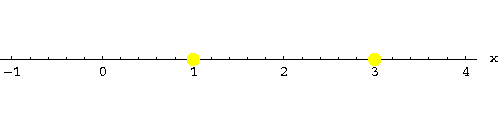Step 2. Our solutions partition the x-axis into three intervals. Pick a point (your choice!) in each interval. Let me take x=0,x=2 and x=4. Compute f(x) for these points: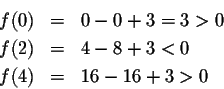These three points are representative for what happens in the intervals they are contained in:

Since f(0)>0, f(x) will be positive for all x in the interval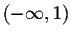. Similarly, since f(2)<0, f(x) will be negative for all x in the interval (1,3). Since f(4)>0, f(x) will be positive for all x in the interval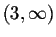. You can indicate this on the x-axis by inserting plus or minus signs on the x-axis. I use color coding instead: blue for positive, red for negative: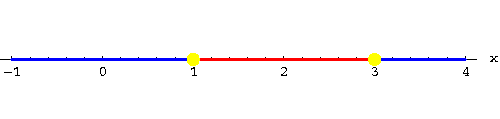Step 3. We want to solve the inequality

x2-4x+3<0,

so we are looking for all x such that f(x)<0. Consequently, the interval (1,3) contains all solutions to the inequality.

Why does this work? Let's look at the graph of f(x):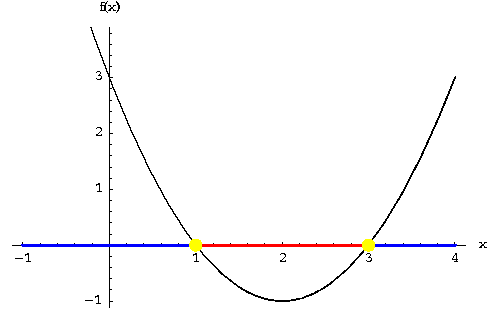Note the pivotal role played by the "yellow dots", the x-intercepts of f(x).

f(x) can only change its sign by passing through an x-intercept, i.e., a solution of f(x)=0 will always separate parts of the graph of f(x) above the x-axis from parts below the x-axis. Thus it suffices to pick a representative in each of the three intervals separated by "yellow dots", to test whether f(x) is positive or negative in the interval .

This nice property of polynomials is called the Intermediate Value Property of polynomials; your teacher might also refer to this property as continuity.

Here is another example: Find the solutions of the inequality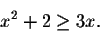For our method to work it is essential that the right side of the inequality equals zero! So let's change our inequality to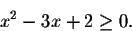Step 1. Solve the equation f(x)=x2-3x+2=0.

Again, we can "factor by guessing":

x2-3x+2=(x-1)(x-2),

so the roots of the equation f(x)=0 are x=1 and x=2. Draw a picture of the x-axis and mark these points.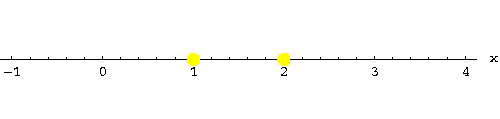Step 2. Our solutions partition the x-axis into three intervals. Pick a point (your choice!) in each interval. Let me take x=0,x=1.5 and x=3. Compute f(x) for these points: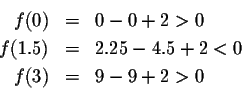These three points are representative for what happens in the intervals they are contained in:

Since f(0)>0, f(x) will be positive for all x in the interval. Similarly, since f(1.5)<0, f(x) will be negative for all x in the interval (1,2). Since f(3)>0, f(x) will be positive for all x in the interval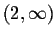. You can indicate this on the x-axis by inserting plus or minus signs on the x-axis. I use color coding instead: blue for positive, red for negative: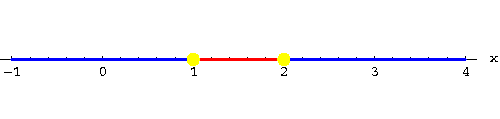Step 3. We want to solve the inequality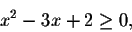so we are looking for all x such that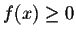. Consequently, the set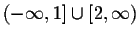contains all solutions to the inequality. (Since our inequality only stipulates that, x=1 and x=2 are solutions, so we include them. "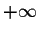" and "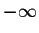" are only symbols; they will never be included as solutions.)

Our next example: Solve x3>2x. Do not divide by x on both sides! If you do so, you will never be able to arrive at the correct answer. Repeat the pattern instead; make one side of the inequality equal zero:

x3-2x>0.

Step 1. Solve the equation f(x)=x3-2x=0.

We can factor rather easily: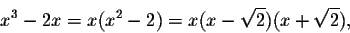so the roots of the equation f(x)=0 are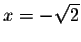, x=0 and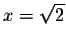. Draw a picture of the x-axis and mark these points.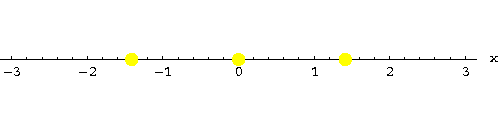Step 2. Our solutions partition the x-axis into four intervals. Pick a point (your choice!) in each interval. Let me take x=-2,x=-1, x=1 and x=2. Compute f(x) for these points: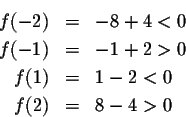These four points are representative for what happens in the intervals they are contained in:

Since f(-2)<0, f(x) will be negative for all x in the interval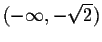. Similarly, since f(-1)>0, f(x) will be positive for all x in the interval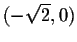. Since f(1)<0, f(x) will be negative for all x in the interval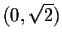. Since f(2)>0, f(x) will be positive for all x in the interval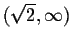. You can indicate this on the x-axis by inserting plus or minus signs on the x-axis. I use color coding instead: blue for positive, red for negative: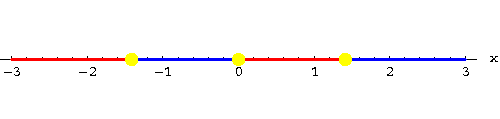Step 3. We want to solve the inequality

x3-2x> 0,

so we are looking for all x such that f(x)> 0. Consequently, the set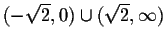contains all solutions to the inequality. (Since our inequality stipulates that f(x)>0,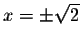do not qualify as solutions, so we exclude them. "" and "" are only symbols; they will never be included as solutions.)

Here is my last example: Solve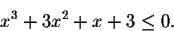Step 1. Solve the equation f(x)=x3+3x2+x+3.

We can factor either by finding a rational zero, or by clever grouping:

x3+3x2+x+3=(x3+3x2)+(x+3)=x2(x+3)+1 (x+3)=(x2+1)(x+3),

so there is only one real root of the equation f(x)=0, namely x=-3. Draw a picture of the x-axis and mark this point.

The polynomial x2+1 is irreducible, it does not have real roots. Its complex roots are irrelevant for our purposes.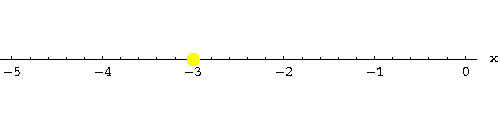Step 2. Our solution partitions the x-axis into two intervals. Pick a point (your choice!) in each interval. Let me take x=-4 and x=0. Compute f(x) for these points: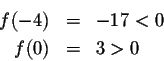These two points are representative for what happens in the intervals they are contained in:

Since f(-4)<0, f(x) will be negative for all x in the interval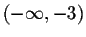. Similarly, since f(0)>0, f(x) will be positive for all x in the interval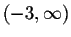. You can indicate this on the x-axis by inserting plus or minus signs on the x-axis. I use color coding instead: blue for positive, red for negative: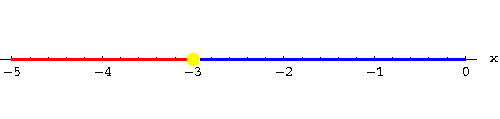Step 3. We want to solve the inequality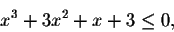so we are looking for all x such that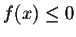. Consequently, the set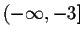is the set of solutions to the inequality. (Since our inequality stipulates that, x=-3 is also a solution, so we include it. "" and "" are only symbols; they will never be included as solutions.)

Time for you to try it yourself:

#### Exercise 1.

Find the solutions of the inequality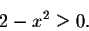#### Exercise 2.

Find the solutions of the inequality

x3<x.

#### Exercise 3.

Find the solutions of the inequality

x3+x2-6x>0.

#### Exercise 4.

Find the solutions of the inequality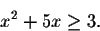#### Exercise 5.

Find the solutions of the inequality[Back] [Next]
[Algebra] [Trigonometry] [Complex Variables]
[Calculus] [Differential Equations] [Matrix Algebra]S.O.S MATHematics home page

Do you need more help? Please post your question on our S.O.S. Mathematics CyberBoard.Helmut Knaust